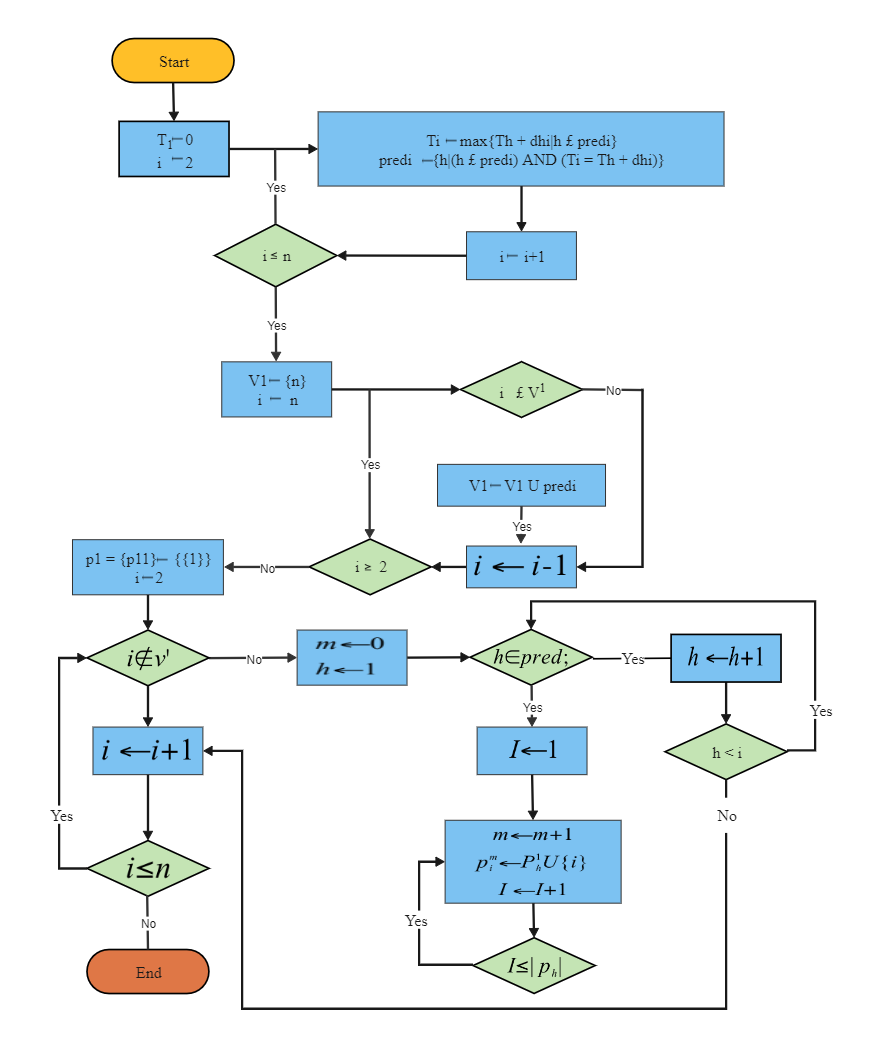Template Community / Predict System Algorithm Flowchart

# Predict System Algorithm FlowchartCharlotte
Published on 2021-07-16
Edit OnlineAn algorithm is a set of rules for carrying out calculations either by hand or on a machine. As suggested below, a finite set of instructions that specify a sequence of operations to be carried out to solve a problem or class of problems can be illustrated by creating an algorithm diagram in EdrawMax. As the below image suggests, an algorithm can be created to understand imaginary numbers. It should be noted here that an imaginary number is a complex number that can be written as an actual number multiplied by the imaginary unit i, which is defined by its property i2= -1. The square of an imaginary number bi is -b2 and others.
Tag
algorithm flowchart
Flowchart Collection
Report
0
97PostRecommended Templates